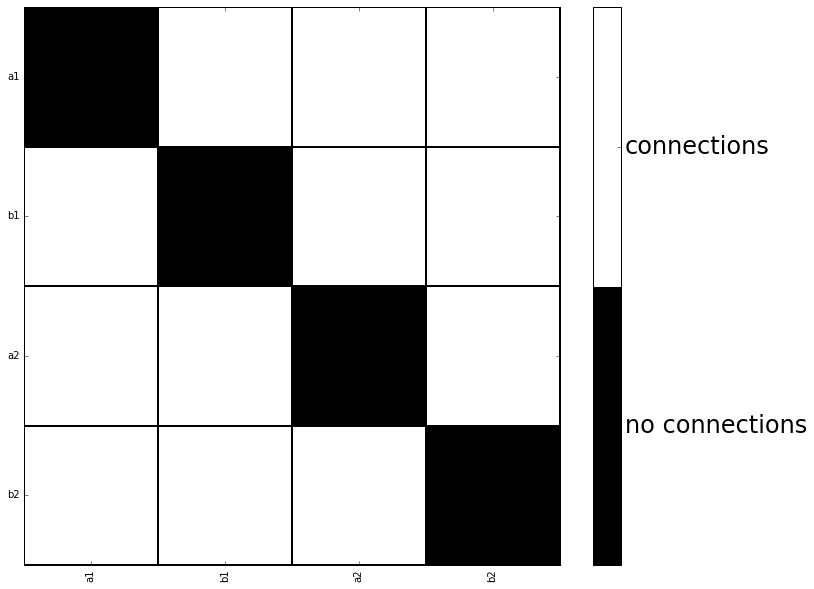In :
%pylab inline
from tvb.simulator.lab import *

Populating the interactive namespace from numpy and matplotlib


Generate surrogate connectivity¶

Testing specific hypotheses about inter-node interactions can often be simpler with just a handful of nodes and connectivity created by hand. TVB makes it simple to construct common scenarios.

In :
# Create empty connectivity
wm = connectivity.Connectivity()

# First weights and distances
nor = 4
wm.motif_all_to_all(number_of_regions=nor)

# Centers, specify the number of regions, otherwise it'll use a default value.
wm.centres_spherical(number_of_regions=nor)

# By default, the new regions labels are numeric characters, ie [0, 1, ...]
wm.create_region_labels(mode='alphabetic')

# But custom region labels can be used
wm.region_labels = ['a1', 'b1', 'a2', 'b2']
wm.configure()

plot_matrix(wm.weights, connectivity=wm, binary_matrix=True)In [ ]: